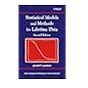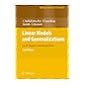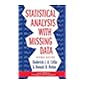Normal view

# Statistical analysis with missing data / Roderick J.A. Little, Donald B. Rubin.

Material type:TextLanguage: English Publisher: Hoboken, N.J. : Wiley, c2002Edition: 2nd edDescription: xv, 381 p. : ill. ; 25 cmISBN: 0471183865 (acidfree paper); 9780471183860DDC classification: 519.5 LOC classification: QA276 | .L57 2002
Contents:
Table of contents The Problem of Missing Data -- Missing-Data Patterns -- Mechanisms That Lead to Missing Data -- A Taxonomy of Missing-Data Methods -- Missing Data in Experiments -- The Exact Least Squares Solution with Complete Data -- The Correct Least Squares Analysis with Missing Data -- Filling in Least Squares Estimates -- Bartlett's ANCOVA Method -- Least Squares Estimates of Missing Values by ANCOVA Using Only Complete-Data Methods -- Correct Least Squares Estimates of Standard Errors and One Degree of Freedom Sums of Squares -- Correct Least Squares Sums of Squares with More Than One Degree of Freedom -- Complete-Case and Available-Case Analysis, Including Weighting Methods -- Complete-Case Analysis -- Weighted Complete-Case Analysis -- Available-Case Analysis -- Single Imputation Methods -- Imputing Means from a Predictive Distribution -- Imputing Draws from a Predictive Distribution -- Estimation of Imputation Uncertainty -- Imputation Methods that Provide Valid Standard Errors from a Single Filled-in Data Set -- Standard Errors for Imputed Data by Resampling -- Introduction to Multiple Imputation -- Comparison of Resampling Methods and Multiple Imputation -- Likelihood-Based Approaches to the Analysis of Missing Data -- Theory of Inference Based on the Likelihood Function -- Review of Likelihood-Based Estimation for Complete Data -- Likelihood-Based Inference with Incomplete Data -- A Generally Flawed Alternative to Maximum Likelihood: Maximizing Over the Parameters and the Missing Data -- Likelihood Theory for Coarsened Data. Factored likelihood methods, ignoring the missing-data mechanism -- Bivariate normal data with one variable subject to nonresponse : ML estimation -- Bivariate normal monotone data : small-sample inference -- monotone data with more than two variables -- Factorizations for special nonmonotone patterns -- Maximum likelihood for general patterns of missing data : introduction and theory with ignorable nonresponse -- Alternative computational strategies -- Introduction to the EM algorithm -- The E and M steps of EM -- Theory of the EM algorithm -- extensions of EM -- Hybrid maximization methods -- Large-sample inference based on maximum likelihood estimates -- Standard errors based on the information matrix -- Standard errors via methods that do not require computing and inverting an estimate of the observed information matrix -- Bayes and multiple imputation -- Bayesian iterative simulation methods -- Multiple imputation -- Multivariate normal examples, ignoring the missing-data mechanism -- Inference for a mean vector and covariance matrix with missing data under normality -- Estimation with a restricted covariance matrix -- Multiple linear regression -- A general repeated-measures model with missing data -- Time series models -- Robust estimation -- Robust estimation for a univariate sample -- Robust estimation of the mean and covariance matrix -- Further extensions of the t model. Models for partially classified contingency tables, ignoring the missing-data mechanism -- Factored likelihoods for monotone multinomial data -- ML and Bayes estimation for multinomial samples with general patterns of missing data -- Loglinear models for partially classified contingency tables -- mixed normal and non-normal data with missing values, ignoring the missing-data mechanism -- The general location model -- The general location model with parameter constraints -- Regression problems involving mixtures of continuous and categorical variables -- Futher extensions of the general location model -- Nonignorable missing-data models -- Likelihood theory for nonignorable models -- Models with known nonignorable missing-data mechanisms : grouped and rounded data -- Normal selection models -- Normal pattern-mixture models -- Nonignorable models for normal repeated-measures data -- Nonignorable models for categorical data.
Summary: Summary: Incorporating a large body of new work in the field, this work includes the applications of modern missing data methods to real data. It also examines the theoretical and technical extensions that take advantage of computational advances.
Tags from this library: No tags from this library for this title.Average rating: 0.0 (0 votes)
Item type Current location Collection Call number Copy number Status Date due Barcode Item holdsE-Book
E-book
Non-fiction 519.5 LIS 2002 (Browse shelf) Not For LoanText
Reserve Section
Non-fiction 519.5 LIS 2002 (Browse shelf) C-1 Not For Loan 25711
Total holds: 0
##### Browsing EWU Library shelves, Shelving location: E-book Close shelf browser519.5 LAS 2003 Statistical models and methods for lifetime data / 519.5 LIB 1994 Basic statistics for business and economics / 519.5 LIN 2008 Linear models and generalizations : 519.5 LIS 2002 Statistical analysis with missing data / 519.5 LIS 2012 Statistical techniques in business & economics / 519.5 LIS 2012 Statistical techniques in business & economics / 519.5 LIS 2018 Statistical techniques in business & economics /

Online version:
Little, Roderick J.A.
Statistical analysis with missing data.
Hoboken, N.J. : Wiley, c2002
(OCoLC)645898508

Includes bibliographical references (p. 349-364) and indexes.

Missing-Data Patterns --
Mechanisms That Lead to Missing Data --
A Taxonomy of Missing-Data Methods --
Missing Data in Experiments --
The Exact Least Squares Solution with Complete Data --
The Correct Least Squares Analysis with Missing Data --
Filling in Least Squares Estimates --
Bartlett's ANCOVA Method --
Least Squares Estimates of Missing Values by ANCOVA Using Only Complete-Data Methods --
Correct Least Squares Estimates of Standard Errors and One Degree of Freedom Sums of Squares --
Correct Least Squares Sums of Squares with More Than One Degree of Freedom --
Complete-Case and Available-Case Analysis, Including Weighting Methods --
Complete-Case Analysis --
Weighted Complete-Case Analysis --
Available-Case Analysis --
Single Imputation Methods --
Imputing Means from a Predictive Distribution --
Imputing Draws from a Predictive Distribution --
Estimation of Imputation Uncertainty --
Imputation Methods that Provide Valid Standard Errors from a Single Filled-in Data Set --
Standard Errors for Imputed Data by Resampling --
Introduction to Multiple Imputation --
Comparison of Resampling Methods and Multiple Imputation --
Likelihood-Based Approaches to the Analysis of Missing Data --
Theory of Inference Based on the Likelihood Function --
Review of Likelihood-Based Estimation for Complete Data --
Likelihood-Based Inference with Incomplete Data --
A Generally Flawed Alternative to Maximum Likelihood: Maximizing Over the Parameters and the Missing Data --
Likelihood Theory for Coarsened Data. Factored likelihood methods, ignoring the missing-data mechanism --
Bivariate normal data with one variable subject to nonresponse : ML estimation --
Bivariate normal monotone data : small-sample inference --
monotone data with more than two variables --
Factorizations for special nonmonotone patterns --
Maximum likelihood for general patterns of missing data : introduction and theory with ignorable nonresponse --
Alternative computational strategies --
Introduction to the EM algorithm --
The E and M steps of EM --
Theory of the EM algorithm --
extensions of EM --
Hybrid maximization methods --
Large-sample inference based on maximum likelihood estimates --
Standard errors based on the information matrix --
Standard errors via methods that do not require computing and inverting an estimate of the observed information matrix --
Bayes and multiple imputation --
Bayesian iterative simulation methods --
Multiple imputation --
Multivariate normal examples, ignoring the missing-data mechanism --
Inference for a mean vector and covariance matrix with missing data under normality --
Estimation with a restricted covariance matrix --
Multiple linear regression --
A general repeated-measures model with missing data --
Time series models --
Robust estimation --
Robust estimation for a univariate sample --
Robust estimation of the mean and covariance matrix --
Further extensions of the t model. Models for partially classified contingency tables, ignoring the missing-data mechanism --
Factored likelihoods for monotone multinomial data --
ML and Bayes estimation for multinomial samples with general patterns of missing data --
Loglinear models for partially classified contingency tables --
mixed normal and non-normal data with missing values, ignoring the missing-data mechanism --
The general location model --
The general location model with parameter constraints --
Regression problems involving mixtures of continuous and categorical variables --
Futher extensions of the general location model --
Nonignorable missing-data models --
Likelihood theory for nonignorable models --
Models with known nonignorable missing-data mechanisms : grouped and rounded data --
Normal selection models --
Normal pattern-mixture models --
Nonignorable models for normal repeated-measures data --
Nonignorable models for categorical data.

Summary:
Incorporating a large body of new work in the field, this work includes the applications of modern missing data methods to real data. It also examines the theoretical and technical extensions that take advantage of computational advances.

Applied Statistics

There are no comments for this item.